19.2
19.1
18.2
18.1
17.2

# Totals

The Grid dashboard item enables you to add a summary value (a total) calculated against displayed values of an individual column, and to show the result under this column.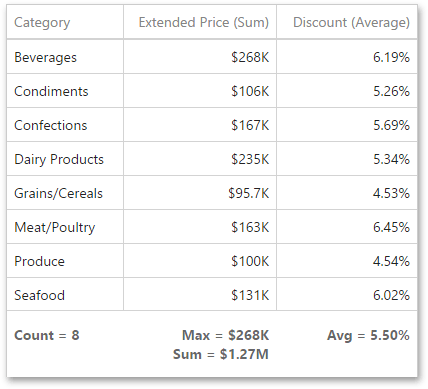Note that you can add any number of totals for each column. For example, you can obtain the number of column records, average or maximum value, etc.

## Totals Overview

You can use the following summary functions when creating totals.

 Count The number of records. Sum The sum of the values.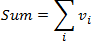Min The smallest value. Max The largest value. Avg The average of the values.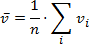Auto The total is calculated using the type of summary function specified for the measure corresponding to the current Grid column. Note that in this case, the total is calculated based on values of the corresponding data field from the underlying data source.

You can calculate totals for all types of grid columns (GridColumn descendants), but each column type supports only a specified set of summary functions used to calculate totals. The table below lists the total types supported by different grid columns.

Column Type

Supported Total Types

GridMeasureColumn

Auto

Avg

Count

Max

Min

Sum

GridDimensionColumn

Min

Max

Count

GridDeltaColumn

Count

GridSparklineColumn

Count

Totals, except the Auto type, are calculated based on summary values. When the GridColumnTotal.totalType property is set to Auto, the total is calculated based on values of the corresponding data field from the underlying data source. In this case, the Measure.summaryType property value is taken into account to calculate the total. If the specified summaryType type is not matched with supported total types, the Count summary function is used.

## Create and Edit Totals in UI

To create a total, open a data item menu and go to the Totals section. Click "+" to add a new total.

You can change the total type by opening the drop down list and selecting the required type.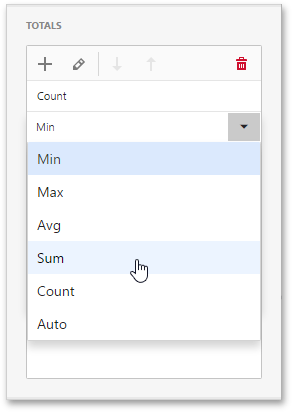To delete the total, click the Delete button (the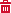icon).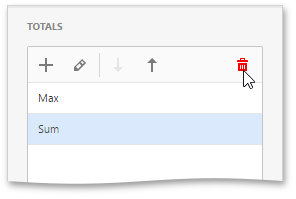## Create and Edit Totals in code

The Grid dashboard item allows you to calculate totals against values displayed in the specified column and show these totals under the column. To create a total for the required column, do the following.